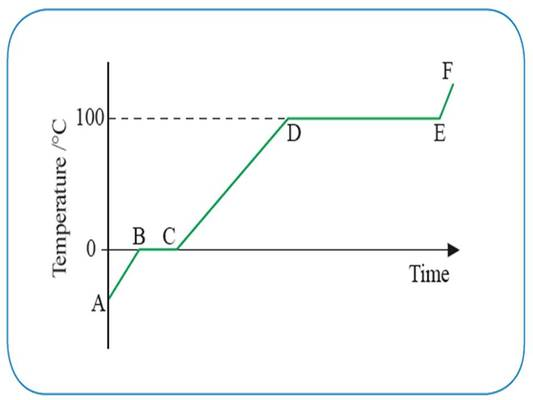Temperature & Solids Liquids and Gasses
3 years ago
lisahsfc
Save
Edit
Host a game
Live GameLive
Homework
Solo Practice
Practice15 QuestionsShow answers
• Question 1
30 seconds
Q. Which of these is not true for solids?
Particles can move freely
Particles vibrate about a fixed position
Particles are held by strong inter-molecular forces
Particles are regularly arranged
• Question 2
30 seconds
Q. Which of these is true for a liquid?
Particles can move freely
Particles vibrate about a fixed position
Particles are held by strong inter-molecular forces
Particles are regularly arranged
• Question 3
30 seconds
Q. Which of these is NOT true for a liquid
Particles are randomly arranged
Particles can move freely
Particles have greater energy
• Question 4
30 secondsQ. Assuming the graph shown is for water which section shows a change of state from solid to liquid?
A-B
B-C
C-D
D-E
• Question 5
30 secondsQ. Assuming the graph shown is for water which section shows a change of state from liquid to gas?
A-B
B-C
C-D
D-E
• Question 6
30 secondsQ. Assuming the graph shown is for water which section shows a change of state from water to steam?
A-B
B-C
C-D
D-E
• Question 7
30 secondsQ. What state will the water be in in section E-F?
Solid
liquid
gas
plasma
• Question 8
30 secondsQ. What state will the water be in in section A-B?
Solid
liquid
gas
plasma
• Question 9
30 secondsQ. What state will the water be in in section C-D?
Solid
liquid
gas
plasma
• Question 10
30 secondsQ. What is happening in section B-C?
A. There is an increase in the kinetic energy of the molecules
B. There is an increase in the electrical potential energy of the molecules
There is a decrease in the electrical potential energy of the molecules
A and B
• Question 11
30 secondsQ. What is happening in section A-B?
A. There is an increase in the kinetic energy of the molecules
B. There is an increase in the electrical potential energy of the molecules
There is a decrease in the electrical potential energy of the molecules
A and B
• Question 12
30 secondsQ. What is happening in section C-D?
A. There is an increase in the kinetic energy of the molecules
B. There is an increase in the electrical potential energy of the molecules
There is a decrease in the electrical potential energy of the molecules
A and B
• Question 13
30 secondsQ. What is happening in section E-F?
A. There is an increase in the kinetic energy of the molecules
B. There is an increase in the electrical potential energy of the molecules
There is a decrease in the electrical potential energy of the molecules
A and B
• Question 14
30 secondsQ. What is happening in section D-E?
A. There is an increase in the kinetic energy of the molecules
B. There is an increase in the electrical potential energy of the molecules
There is a decrease in the electrical potential energy of the molecules
A and B
• Question 15
30 secondsQ. Assuming the graph shown is for water which section shows an increase in temperature?